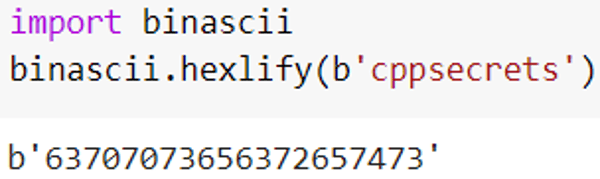Related Tags

python

# What is binascii.hexlify in Python?

binascii is a widely used Python library for ASCII-encoded binary representations. It contains several methods for converting to binary from ASCII or hex, and vice versa.

binascii.hexlify is one of the methods in the binascii library. This method converts the binary representation of the data to hexadecimal. The conversion follows these principles:

1. Every byte is converted to a 2-digit hexadecimal representation.

2. The length of return data is twice the length of input data.

## Syntax

binascii.hexlify(data[, sep[, bytes_per_sep=1]])


## Parameters

1. data: The data that needs to be converted.

2. sep: We use this if a user wants to apply a separator between each converted character.## Code

In this code, we see the working of binascii.hexlify(). First, we convert data to binary format using bytes method. Next, we use binascii.hexlify() to convert the binary data to hexadecimal and print the result. Additionally, we use a separator - to see how the second parameter works.

import binascii

x = "Hello"
res = bytes(x, 'utf-8')

print(binascii.hexlify(res))
print(binascii.hexlify(res , '-'))


RELATED TAGS

python

CONTRIBUTOR